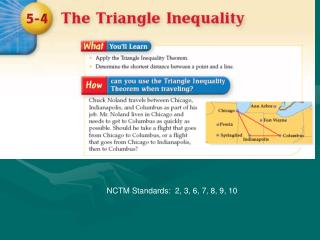DownloadDownload PresentationNCTM Standards: 2, 3, 6, 7, 8, 9, 10

# NCTM Standards: 2, 3, 6, 7, 8, 9, 10

Download Presentation## NCTM Standards: 2, 3, 6, 7, 8, 9, 10

- - - - - - - - - - - - - - - - - - - - - - - - - - - E N D - - - - - - - - - - - - - - - - - - - - - - - - - - -
##### Presentation Transcript

1. NCTM Standards: 2, 3, 6, 7, 8, 9, 10

2. Triangle Inequality Theorem The sum of the lengths of any two sides of a triangle is greater that the length of the third side.

3. Example 4-1a Determine whether the measures and can be lengths of the sides of a triangle. Answer:Because the sum of two measures is not greater than the length of the third side, the sides cannot form a triangle.

4. Example 4-1b Determine whether the measures 6.8, 7.2, and 5.1 can be lengths of the sides of a triangle. Check each inequality. Answer:All of the inequalities are true, so 6.8, 7.2, and 5.1 can be the lengths of the sides of a triangle.

5. Example 4-1c Determine whether the given measures can be lengths of the sides of a triangle. a. 6, 9, 16 b. 14, 16, 27 Answer: no Answer: yes

6. Example 4-2a Multiple-Choice Test ItemIn andWhich measure cannot be PR? A 7 B 9 C 11 D 13

7. Example 4-2a Read the Test Item You need to determine which value is not valid. Solve the Test Item Solve each inequality to determine the range of values for PR.

8. Example 4-2a The range of values that fit all three inequalities is Graph the inequalities on the same number line. Examine the answer choices. The only value that does not satisfy the compound inequality is 13 since 13 is greater than 12.4. Thus, the answer is choice D.

9. Multiple-Choice Test ItemWhich measure cannot be XZ? • 4 • 9 • 12 • 16

10. Distance Between a Point & a Line The perpendicular segment from a point to a line is the shortest segment from the point to the line. All segments can be measured, but PQ is the shortest; it is considered the “distance” between the point and the line. P B R Q Distance is always measured on the perpendicular.. A

11. Corollary The perpendicular segment from a point to a plane is the shortest segment from the point to the plane. All segments can be measured, but PQ is the shortest; it is considered the “distance” between the point and the plane. P B R Q A Distance is always measured on the perpendicular..

12. HW: Page 264